# Frank Solutions for Class 10 Maths Chapter 7 Problems Based On Quadratic Equations

Frank Solutions for Class 10 Maths Chapter 7 Problems Based on Quadratic Equations is the most preferred study material due to its unique description of the concepts. The solutions are designed in an easy-to-comprehend manner to build confidence in students. By practising Frank Solutions for Class 10 Maths, they will develop in-depth knowledge of the concepts and topics covered in this chapter.

Chapter 7 – Problems Based on Quadratic Equations: A quadratic equation is an equation of the second degree, which means that it contains at least one term that is squared. Frank Solutions for Class 10 Maths Chapter 7 contain various methods of solving the equations.

## Download the PDF of Frank Solutions for Class 10 Maths Chapter 7 Problems Based on Quadratic Equations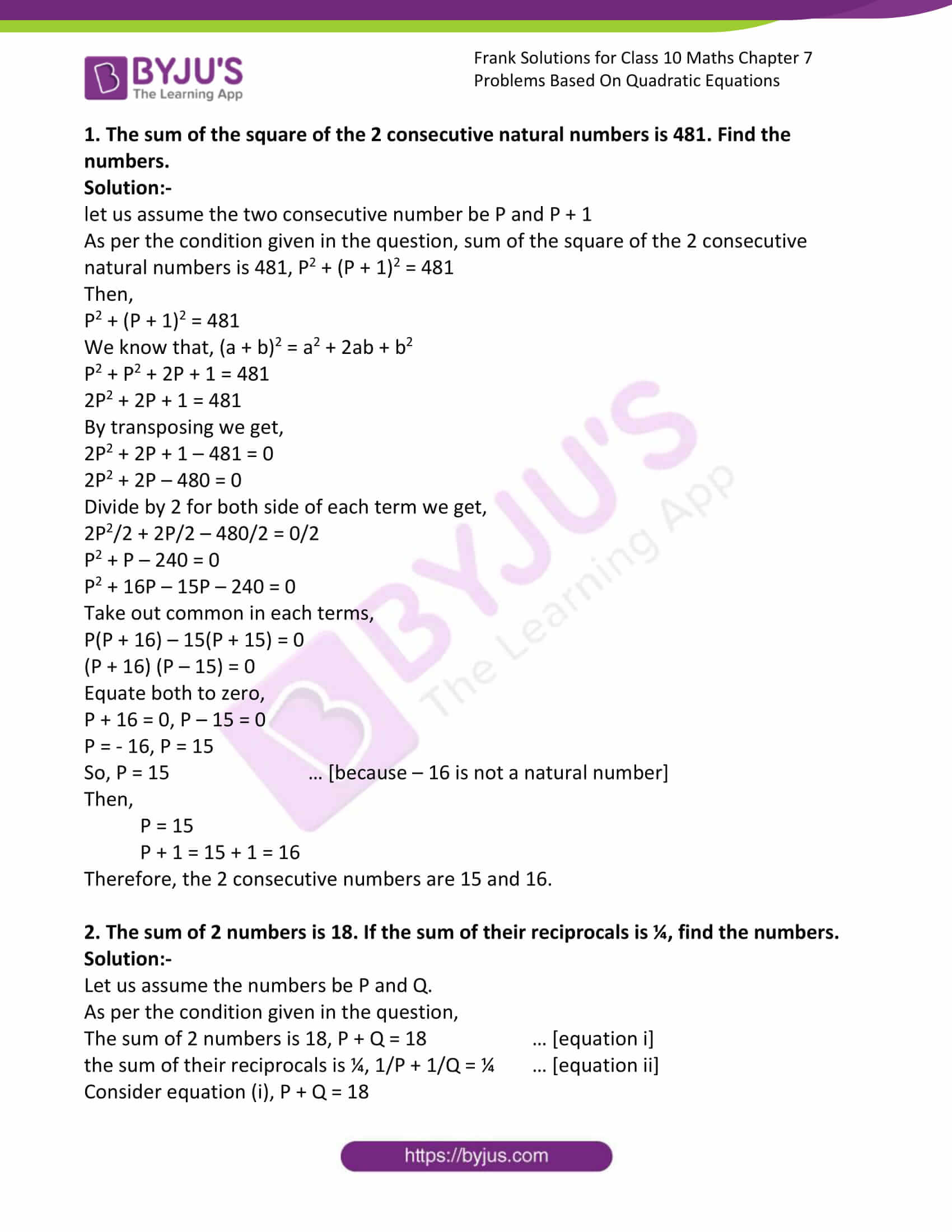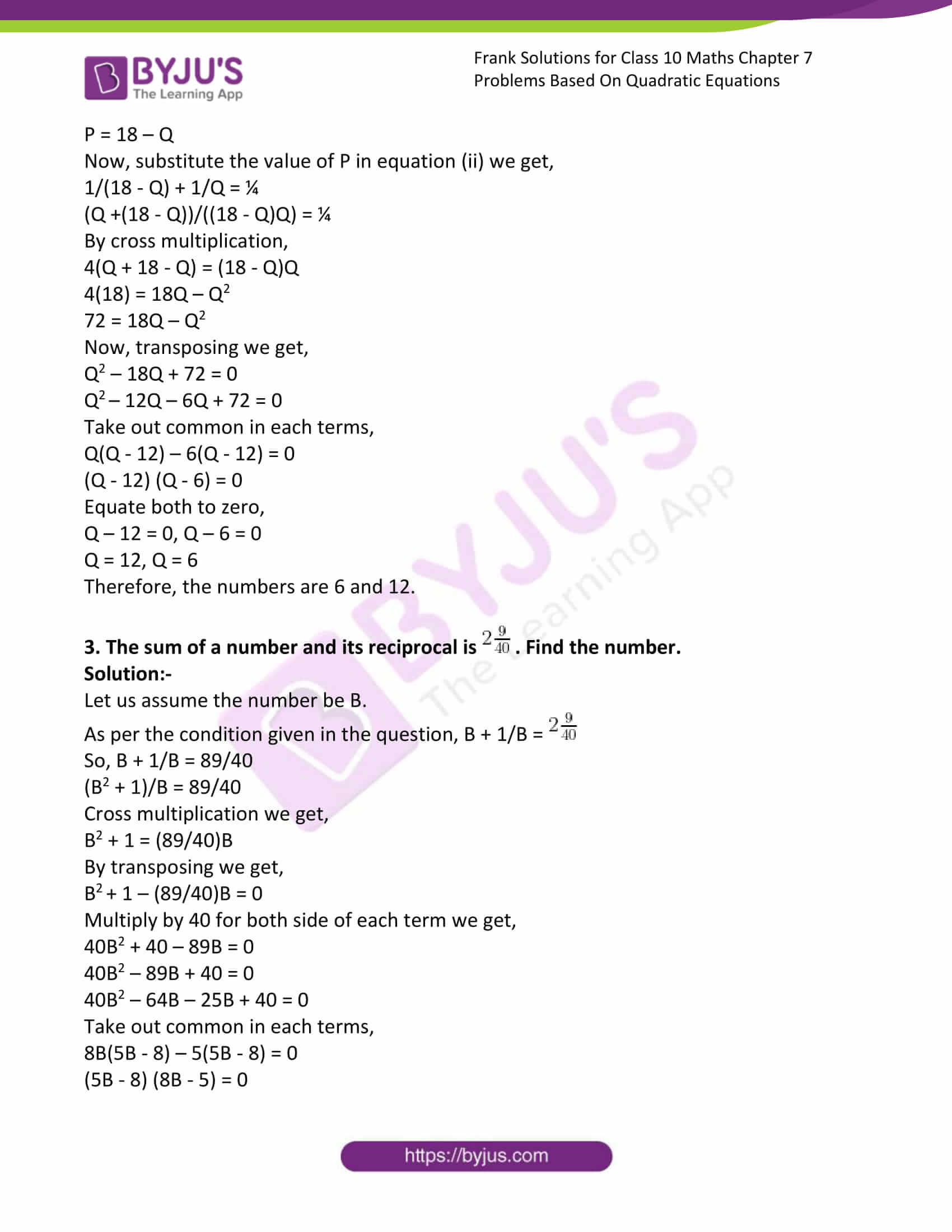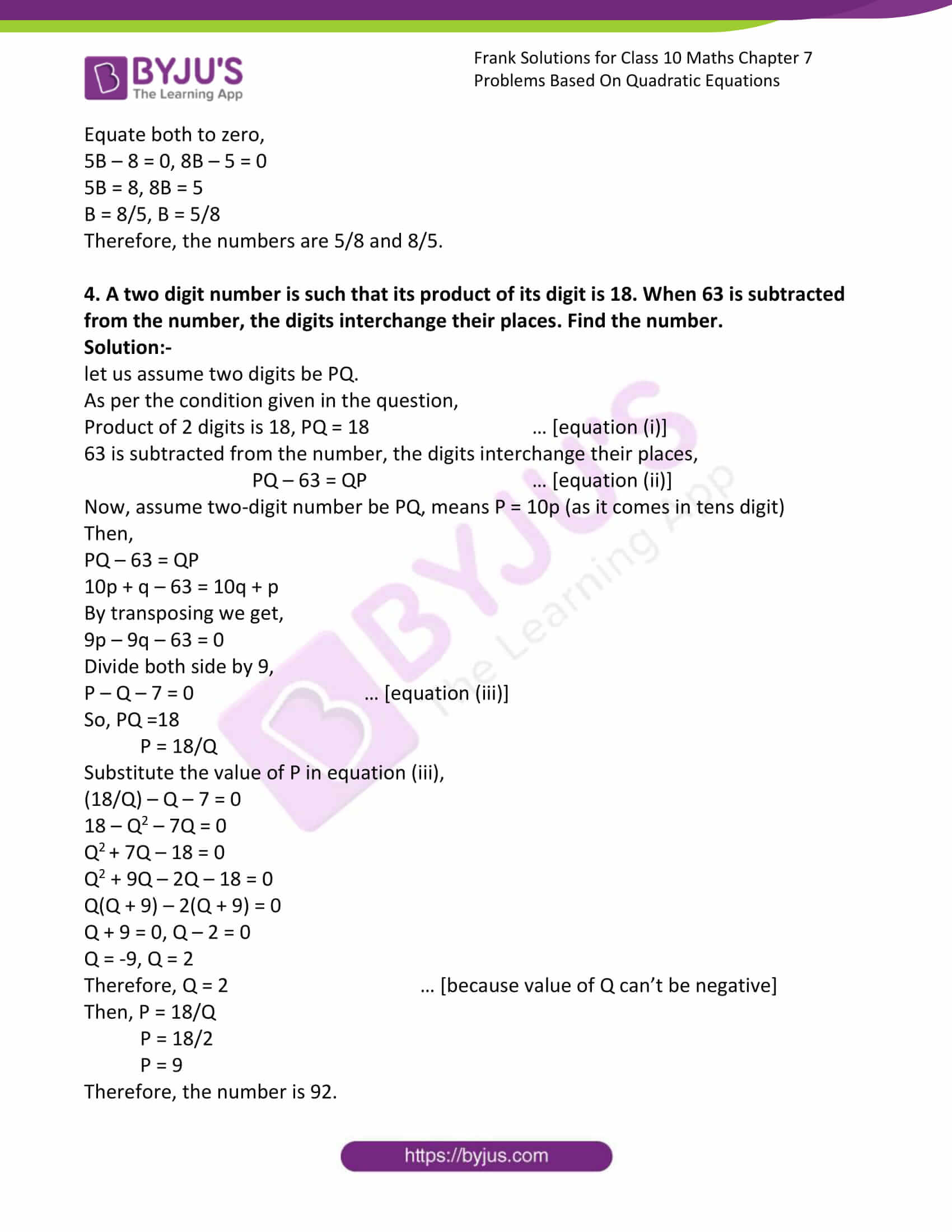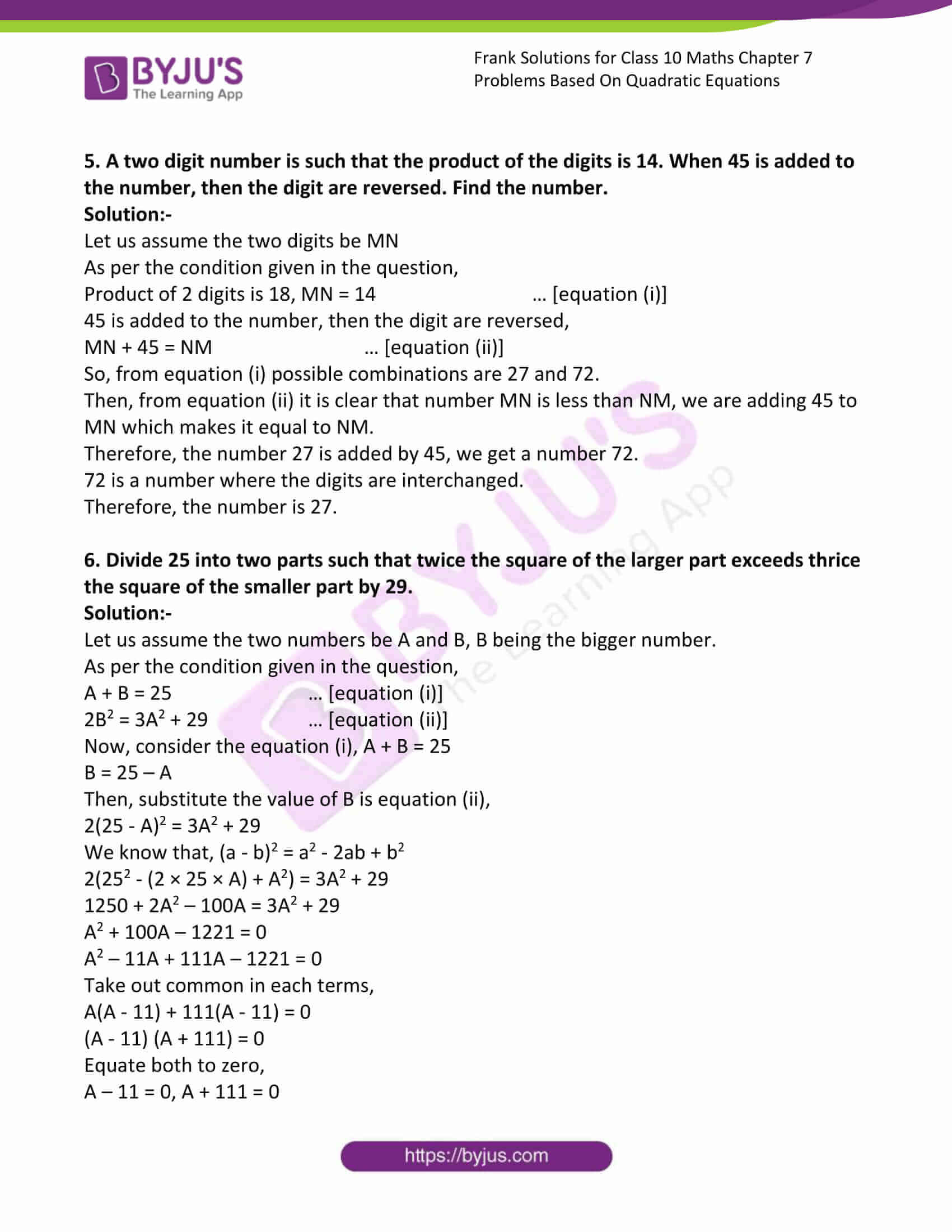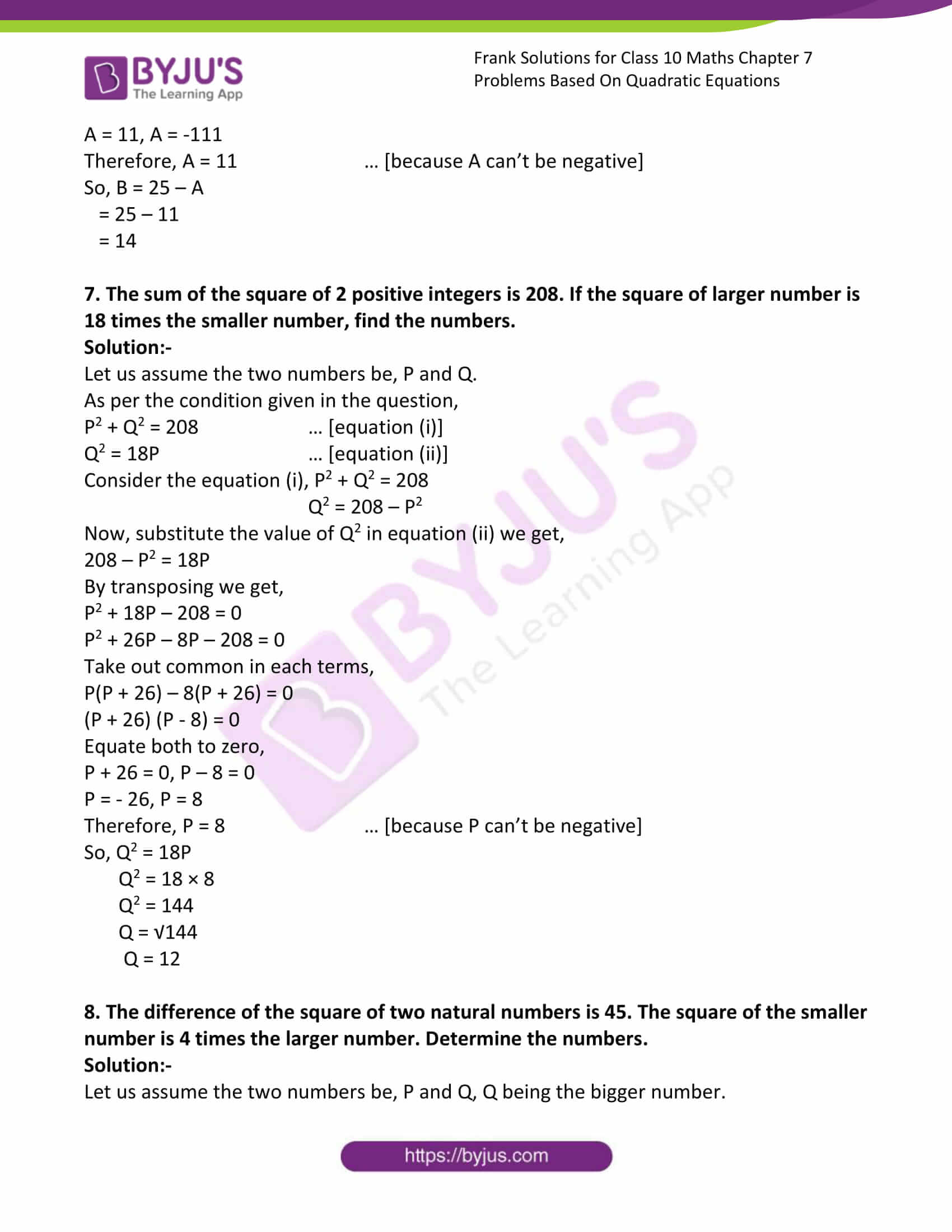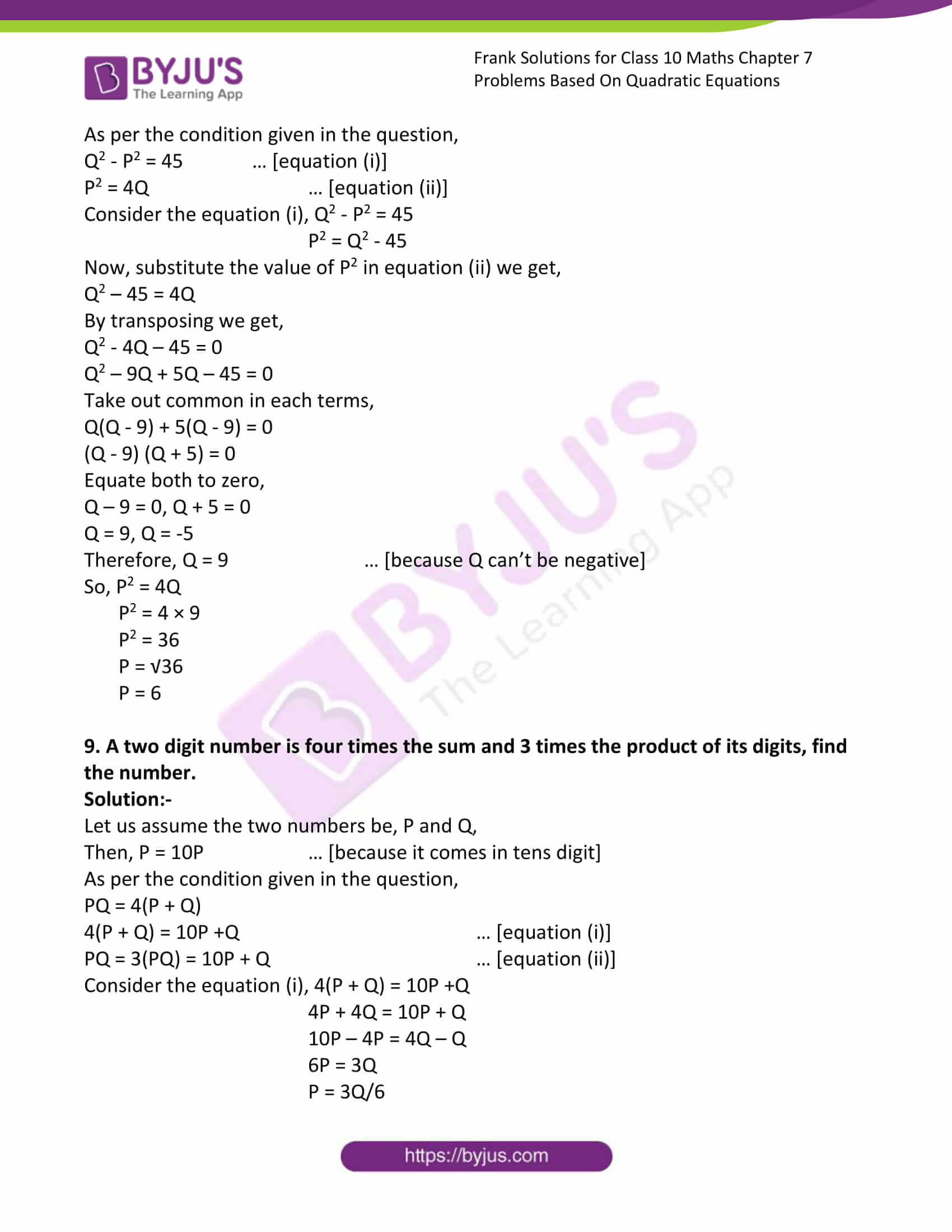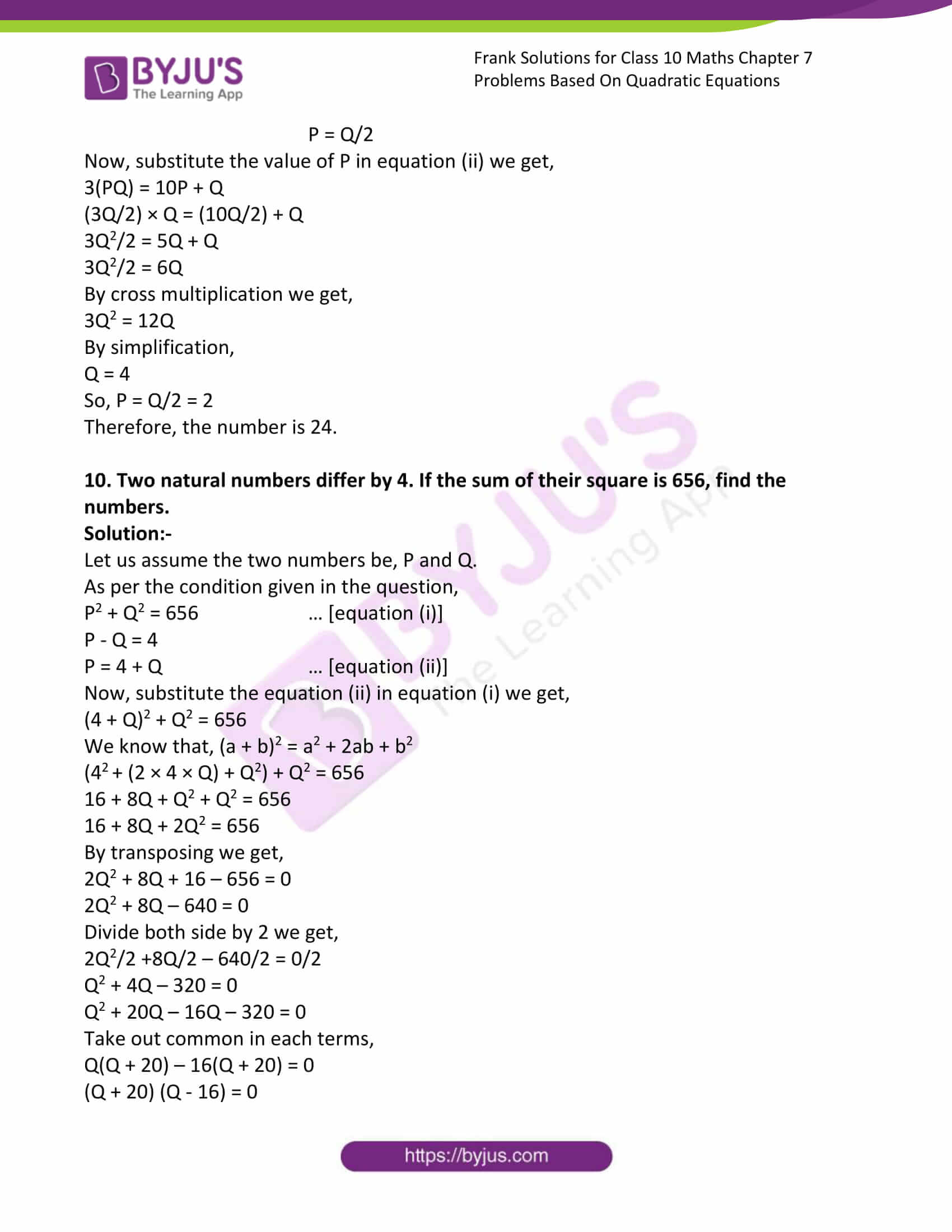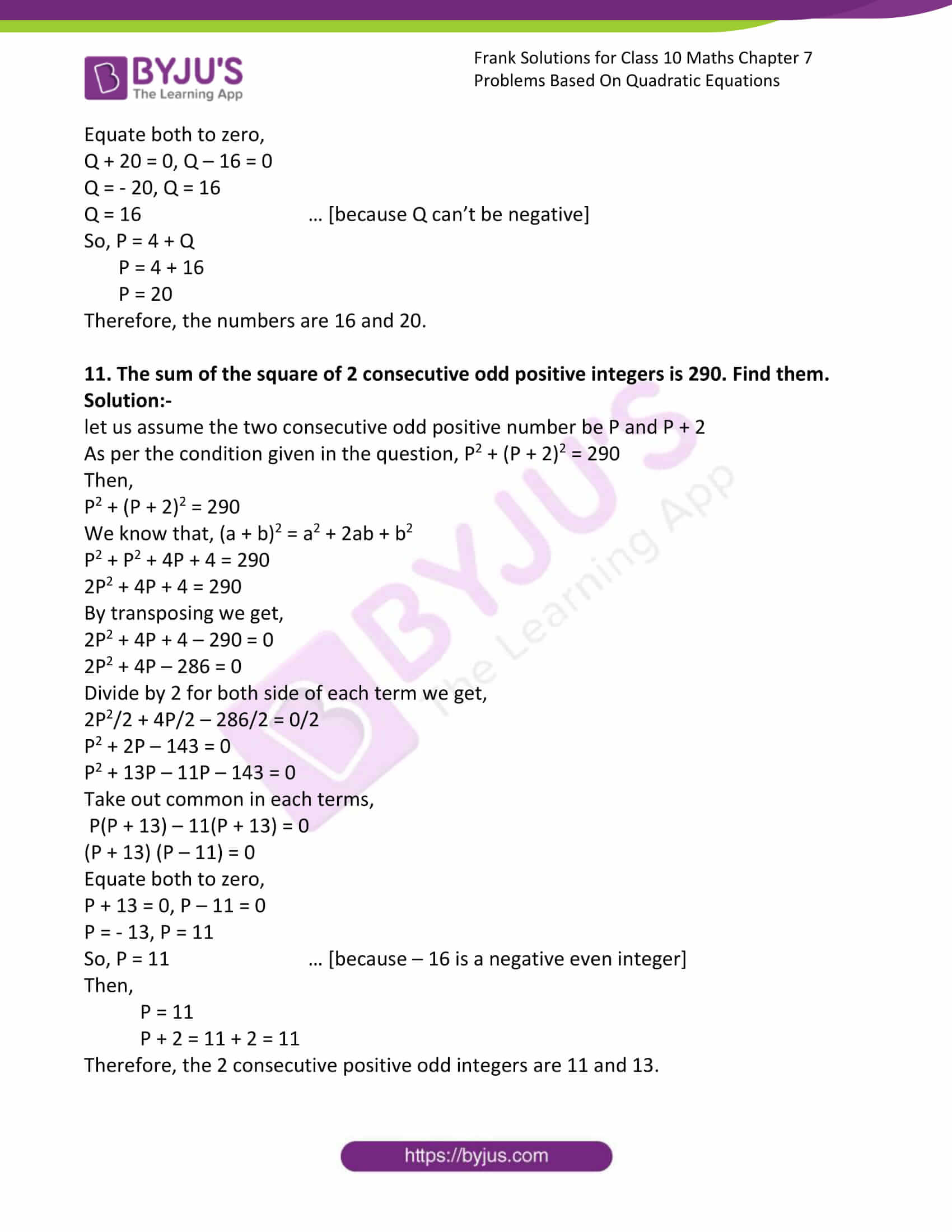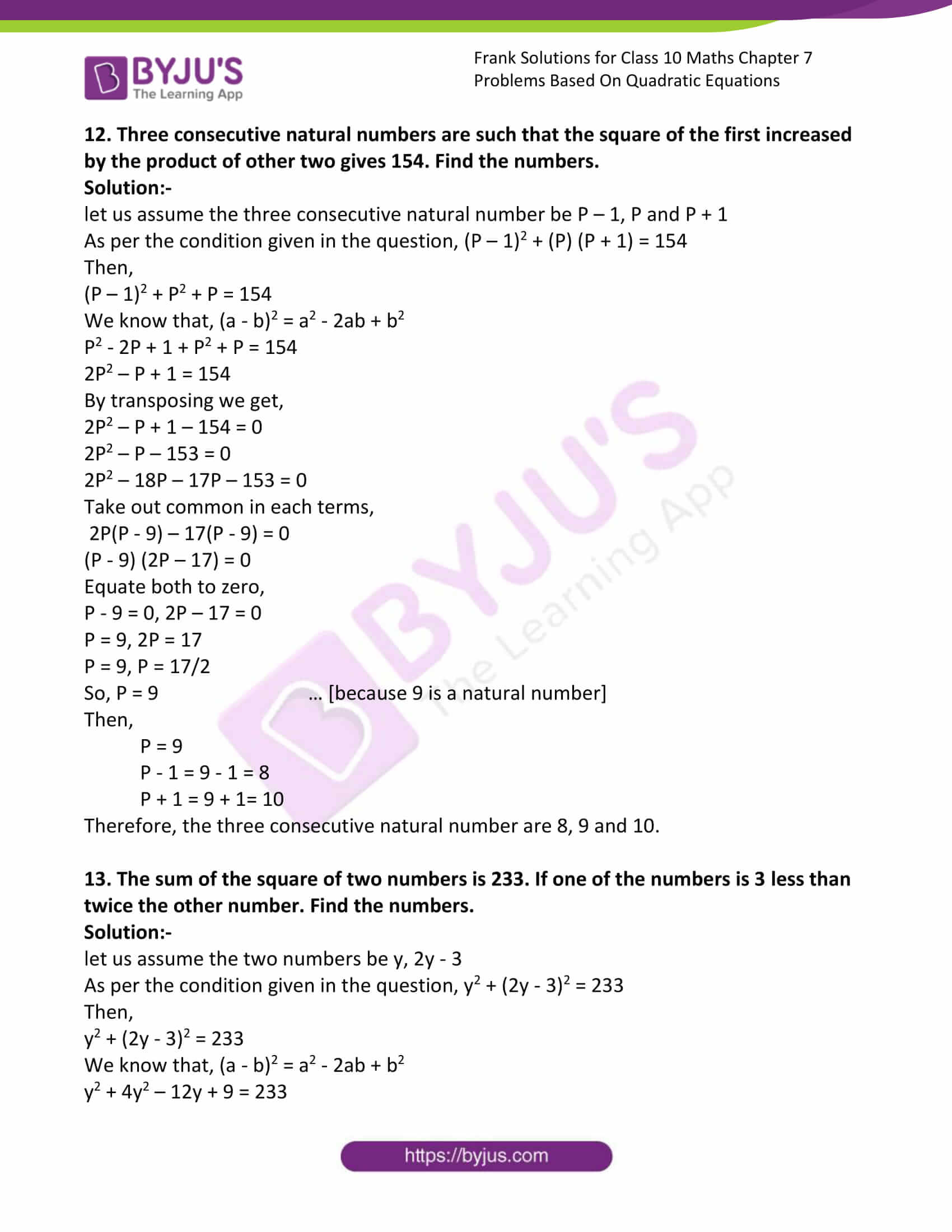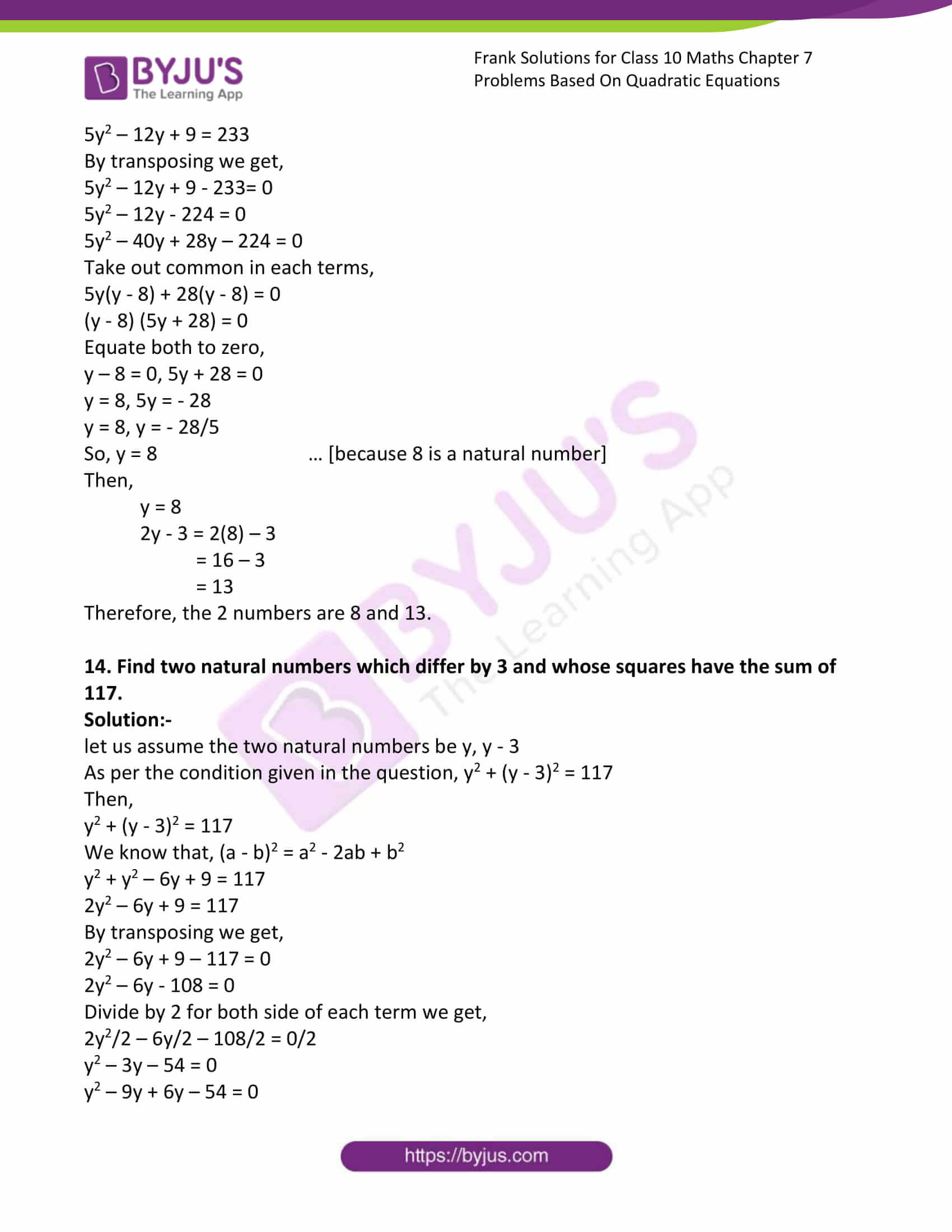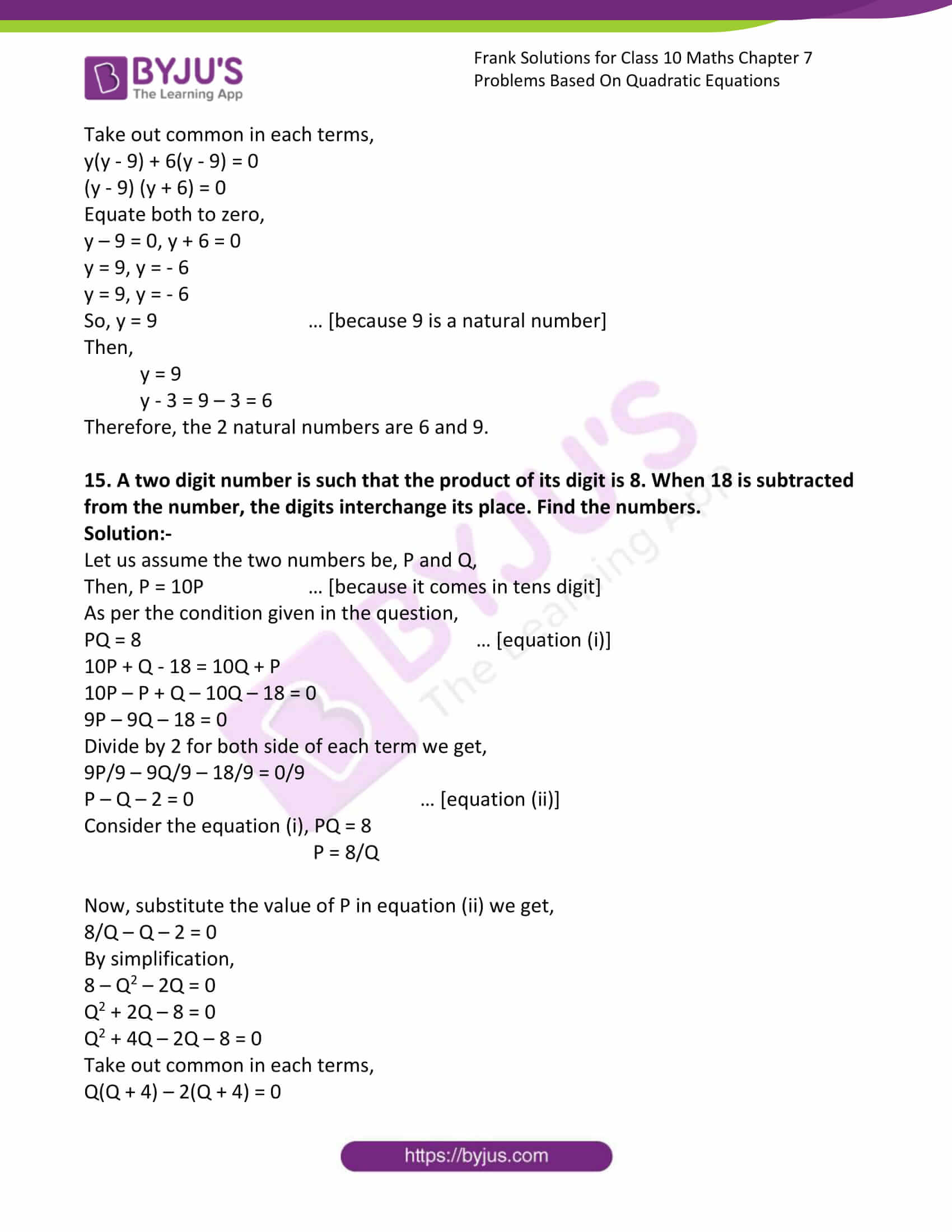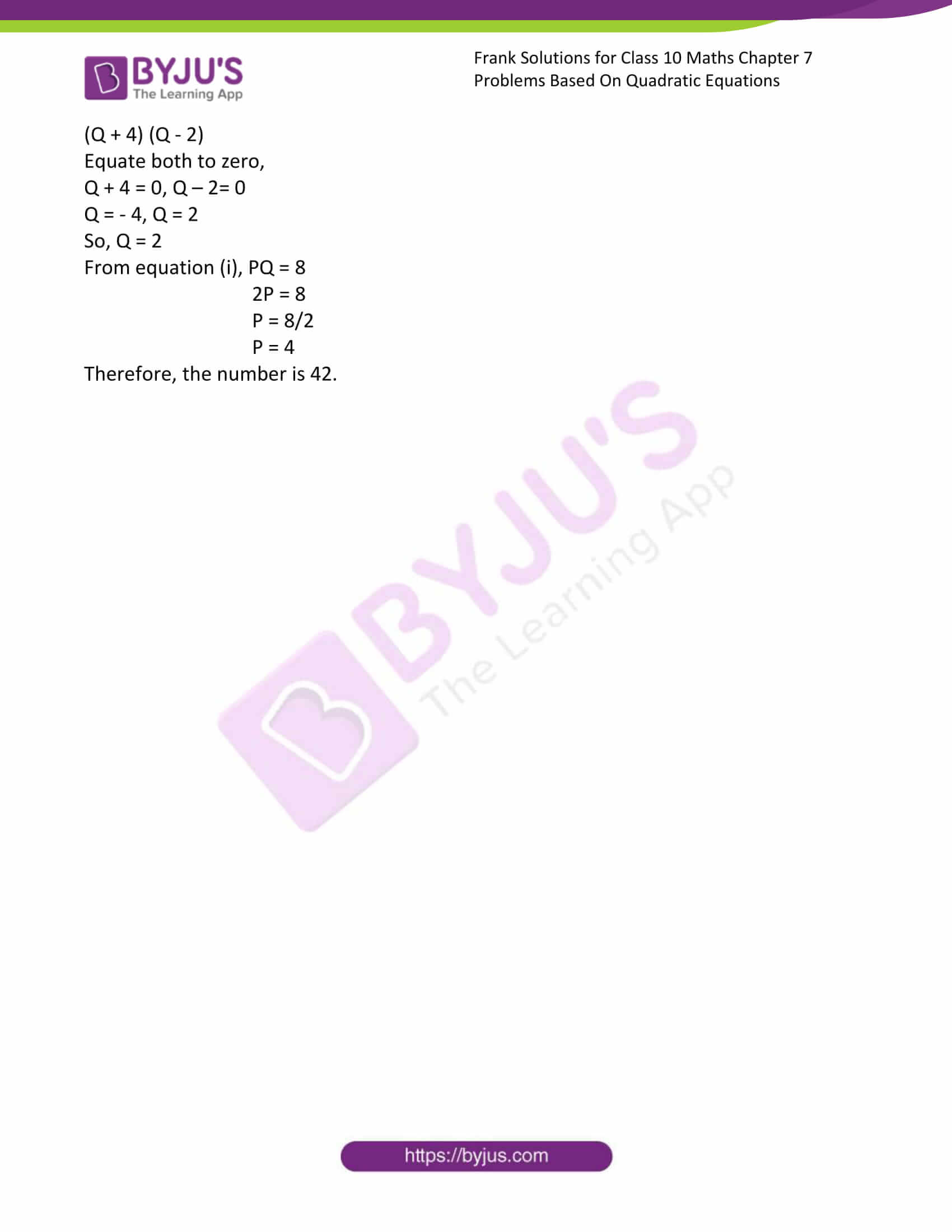### Access Answers to Frank Solutions for Class 10 Maths Chapter 7 Problems Based on Quadratic Equations

1. The sum of the square of the 2 consecutive natural numbers is 481. Find the numbers.

Solution:-

let us assume the two consecutive numbers be P and P + 1

As per the condition given in the question, the sum of the square of the 2 consecutive natural numbers is 481, P2 + (P + 1)2 = 481

Then,

P2 + (P + 1)2 = 481

We know that, (a + b)2 = a2 + 2ab + b2

P2 + P2 + 2P + 1 = 481

2P2 + 2P + 1 = 481

By transposing, we get,

2P2 + 2P + 1 – 481 = 0

2P2 + 2P – 480 = 0

Divide by 2 for both sides of each term we get,

2P2/2 + 2P/2 – 480/2 = 0/2

P2 + P – 240 = 0

P2 + 16P – 15P – 240 = 0

Take out common in each term,

P(P + 16) – 15(P + 15) = 0

(P + 16) (P – 15) = 0

Equate both to zero,

P + 16 = 0, P – 15 = 0

P = – 16, P = 15

So, P = 15 … [because – 16 is not a natural number]

Then,

P = 15

P + 1 = 15 + 1 = 16

Therefore, the 2 consecutive numbers are 15 and 16.

2. The sum of 2 numbers is 18. If the sum of their reciprocals is ¼, find the numbers.

Solution:-

Let us assume the numbers be P and Q.

As per the condition given in the question,

The sum of 2 numbers is 18, P + Q = 18 … [equation i]

the sum of their reciprocals is ¼, 1/P + 1/Q = ¼ … [equation ii]

Consider equation (i), P + Q = 18

P = 18 – Q

Now, substitute the value of P in equation (ii) we get,

1/(18 – Q) + 1/Q = ¼

(Q +(18 – Q))/((18 – Q)Q) = ¼

By cross multiplication,

4(Q + 18 – Q) = (18 – Q)Q

4(18) = 18Q – Q2

72 = 18Q – Q2

Now, transposing, we get,

Q2 – 18Q + 72 = 0

Q2 – 12Q – 6Q + 72 = 0

Take out common in each term,

Q(Q – 12) – 6(Q – 12) = 0

(Q – 12) (Q – 6) = 0

Equate both to zero,

Q – 12 = 0, Q – 6 = 0

Q = 12, Q = 6

Therefore, the numbers are 6 and 12.

3. The sum of a number and its reciprocal is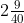. Find the number.

Solution:-

Let us assume the number be B.

As per the condition given in the question, B + 1/B =So, B + 1/B = 89/40

(B2 + 1)/B = 89/40

Cross multiplication, we get,

B2 + 1 = (89/40)B

By transposing, we get,

B2 + 1 – (89/40)B = 0

Multiply by 40 for both sides of each term we get,

40B2 + 40 – 89B = 0

40B2 – 89B + 40 = 0

40B2 – 64B – 25B + 40 = 0

Take out common in each term,

8B(5B – 8) – 5(5B – 8) = 0

(5B – 8) (8B – 5) = 0

Equate both to zero,

5B – 8 = 0, 8B – 5 = 0

5B = 8, 8B = 5

B = 8/5, B = 5/8

Therefore, the numbers are 5/8 and 8/5.

4. A two-digit number is such that the product of its digit is 18. When 63 is subtracted from the number, the digits interchange their places. Find the number.

Solution:-

let us assume two digits be PQ.

As per the condition given in the question,

Product of 2 digits is 18, PQ = 18 … [equation (i)]

63 is subtracted from the number, the digits interchange their places,

PQ – 63 = QP … [equation (ii)]

Now, assume the two-digit number be PQ, which means P = 10p (as it comes in tens digit)

Then,

PQ – 63 = QP

10p + q – 63 = 10q + p

By transposing, we get,

9p – 9q – 63 = 0

Divide both sides by 9,

P – Q – 7 = 0 … [equation (iii)]

So, PQ =18

P = 18/Q

Substitute the value of P in equation (iii),

(18/Q) – Q – 7 = 0

18 – Q2 – 7Q = 0

Q2 + 7Q – 18 = 0

Q2 + 9Q – 2Q – 18 = 0

Q(Q + 9) – 2(Q + 9) = 0

Q + 9 = 0, Q – 2 = 0

Q = -9, Q = 2

Therefore, Q = 2 … [because the value of Q can’t be negative]

Then, P = 18/Q

P = 18/2

P = 9

Therefore, the number is 92.

5. A two-digit number is such that the product of the digits is 14. When 45 is added to the number, then the digit is reversed. Find the number.

Solution:-

Let us assume the two digits be MN

As per the condition given in the question,

Product of 2 digits is 18, MN = 14 … [equation (i)]

45 is added to the number, then the digit is reversed,

MN + 45 = NM … [equation (ii)]

So, from equation (i), possible combinations are 27 and 72.

Then, from equation (ii), it is clear that the number MN is less than NM, we are adding 45 to MN, which makes it equal to NM.

Therefore, the number 27 is added to 45, we get the number 72.

72 is a number where the digits are interchanged.

Therefore, the number is 27.

6. Divide 25 into two parts such that twice the square of the larger part exceeds thrice the square of the smaller part by 29.

Solution:-

Let us assume the two numbers be A and B, B being the bigger number.

As per the condition given in the question,

A + B = 25 … [equation (i)]

2B2 = 3A2 + 29 … [equation (ii)]

Now, consider the equation (i), A + B = 25

B = 25 – A

Then, substitute the value of B is equation (ii),

2(25 – A)2 = 3A2 + 29

We know that, (a – b)2 = a2 – 2ab + b2

2(252 – (2 × 25 × A) + A2) = 3A2 + 29

1250 + 2A2 – 100A = 3A2 + 29

A2 + 100A – 1221 = 0

A2 – 11A + 111A – 1221 = 0

Take out common in each term,

A(A – 11) + 111(A – 11) = 0

(A – 11) (A + 111) = 0

Equate both to zero,

A – 11 = 0, A + 111 = 0

A = 11, A = -111

Therefore, A = 11 … [because A can’t be negative]

So, B = 25 – A

= 25 – 11

= 14

7. The sum of the square of 2 positive integers is 208. If the square of a larger number is 18 times the smaller number, find the numbers.

Solution:-

Let us assume the two numbers be, P and Q.

As per the condition given in the question,

P2 + Q2 = 208 … [equation (i)]

Q2 = 18P … [equation (ii)]

Consider the equation (i), P2 + Q2 = 208

Q2 = 208 – P2

Now, substitute the value of Q2 in equation (ii) we get,

208 – P2 = 18P

By transposing, we get,

P2 + 18P – 208 = 0

P2 + 26P – 8P – 208 = 0

Take out common in each term,

P(P + 26) – 8(P + 26) = 0

(P + 26) (P – 8) = 0

Equate both to zero,

P + 26 = 0, P – 8 = 0

P = – 26, P = 8

Therefore, P = 8 … [because P can’t be negative]

So, Q2 = 18P

Q2 = 18 × 8

Q2 = 144

Q = √144

Q = 12

8. The difference of the square of two natural numbers is 45. The square of the smaller number is 4 times the larger number. Determine the numbers.

Solution:-

Let us assume the two numbers be P and Q, Q being the bigger number.

As per the condition given in the question,

Q2 – P2 = 45 … [equation (i)]

P2 = 4Q … [equation (ii)]

Consider the equation (i), Q2 – P2 = 45

P2 = Q2 – 45

Now, substitute the value of P2 in equation (ii) we get,

Q2 – 45 = 4Q

By transposing, we get,

Q2 – 4Q – 45 = 0

Q2 – 9Q + 5Q – 45 = 0

Take out common in each term,

Q(Q – 9) + 5(Q – 9) = 0

(Q – 9) (Q + 5) = 0

Equate both to zero,

Q – 9 = 0, Q + 5 = 0

Q = 9, Q = -5

Therefore, Q = 9 … [because Q can’t be negative]

So, P2 = 4Q

P2 = 4 × 9

P2 = 36

P = √36

P = 6

9. A two-digit number is four times the sum and 3 times the product of its digits; find the number.

Solution:-

Let us assume the two numbers be, P and Q,

Then, P = 10P … [because it comes in tens digit]

As per the condition given in the question,

PQ = 4(P + Q)

4(P + Q) = 10P +Q … [equation (i)]

PQ = 3(PQ) = 10P + Q … [equation (ii)]

Consider the equation (i), 4(P + Q) = 10P +Q

4P + 4Q = 10P + Q

10P – 4P = 4Q – Q

6P = 3Q

P = 3Q/6

P = Q/2

Now, substitute the value of P in equation (ii) we get,

3(PQ) = 10P + Q

(3Q/2) × Q = (10Q/2) + Q

3Q2/2 = 5Q + Q

3Q2/2 = 6Q

By cross multiplication, we get,

3Q2 = 12Q

By simplification,

Q = 4

So, P = Q/2 = 2

Therefore, the number is 24.

10. Two natural numbers differ by 4. If the sum of their square is 656, find the numbers.

Solution:-

Let us assume the two numbers be, P and Q.

As per the condition given in the question,

P2 + Q2 = 656 … [equation (i)]

P – Q = 4

P = 4 + Q … [equation (ii)]

Now, substitute equation (ii) with equation (i) we get,

(4 + Q)2 + Q2 = 656

We know that, (a + b)2 = a2 + 2ab + b2

(42 + (2 × 4 × Q) + Q2) + Q2 = 656

16 + 8Q + Q2 + Q2 = 656

16 + 8Q + 2Q2 = 656

By transposing, we get,

2Q2 + 8Q + 16 – 656 = 0

2Q2 + 8Q – 640 = 0

Divide both sides by 2, we get,

2Q2/2 +8Q/2 – 640/2 = 0/2

Q2 + 4Q – 320 = 0

Q2 + 20Q – 16Q – 320 = 0

Take out common in each term,

Q(Q + 20) – 16(Q + 20) = 0

(Q + 20) (Q – 16) = 0

Equate both to zero,

Q + 20 = 0, Q – 16 = 0

Q = – 20, Q = 16

Q = 16 … [because Q can’t be negative]

So, P = 4 + Q

P = 4 + 16

P = 20

Therefore, the numbers are 16 and 20.

11. The sum of the square of 2 consecutive odd positive integers is 290. Find them.

Solution:-

let us assume the two consecutive odd positive numbers be P and P + 2

As per the condition given in the question, P2 + (P + 2)2 = 290

Then,

P2 + (P + 2)2 = 290

We know that, (a + b)2 = a2 + 2ab + b2

P2 + P2 + 4P + 4 = 290

2P2 + 4P + 4 = 290

By transposing, we get,

2P2 + 4P + 4 – 290 = 0

2P2 + 4P – 286 = 0

Divide by 2 for both sides of each term we get,

2P2/2 + 4P/2 – 286/2 = 0/2

P2 + 2P – 143 = 0

P2 + 13P – 11P – 143 = 0

Take out common in each term,

P(P + 13) – 11(P + 13) = 0

(P + 13) (P – 11) = 0

Equate both to zero,

P + 13 = 0, P – 11 = 0

P = – 13, P = 11

So, P = 11 … [because – 16 is a negative even integer]

Then,

P = 11

P + 2 = 11 + 2 = 11

Therefore, the 2 consecutive positive odd integers are 11 and 13.

12. Three consecutive natural numbers are such that the square of the first increased by the product of the other two gives 154. Find the numbers.

Solution:-

let us assume the three consecutive natural numbers be P – 1, P and P + 1

As per the condition given in the question, (P – 1)2 + (P) (P + 1) = 154

Then,

(P – 1)2 + P2 + P = 154

We know that, (a – b)2 = a2 – 2ab + b2

P2 – 2P + 1 + P2 + P = 154

2P2 – P + 1 = 154

By transposing, we get,

2P2 – P + 1 – 154 = 0

2P2 – P – 153 = 0

2P2 – 18P – 17P – 153 = 0

Take out common in each term,

2P(P – 9) – 17(P – 9) = 0

(P – 9) (2P – 17) = 0

Equate both to zero,

P – 9 = 0, 2P – 17 = 0

P = 9, 2P = 17

P = 9, P = 17/2

So, P = 9 … [because 9 is a natural number]

Then,

P = 9

P – 1 = 9 – 1 = 8

P + 1 = 9 + 1= 10

Therefore, the three consecutive natural numbers are 8, 9 and 10.

13. The sum of the square of two numbers is 233. If one of the numbers is 3 less than twice the other number. Find the numbers.

Solution:-

let us assume the two numbers be y, 2y – 3

As per the condition given in the question, y2 + (2y – 3)2 = 233

Then,

y2 + (2y – 3)2 = 233

We know that, (a – b)2 = a2 – 2ab + b2

y2 + 4y2 – 12y + 9 = 233

5y2 – 12y + 9 = 233

By transposing, we get,

5y2 – 12y + 9 – 233= 0

5y2 – 12y – 224 = 0

5y2 – 40y + 28y – 224 = 0

Take out common in each term,

5y(y – 8) + 28(y – 8) = 0

(y – 8) (5y + 28) = 0

Equate both to zero,

y – 8 = 0, 5y + 28 = 0

y = 8, 5y = – 28

y = 8, y = – 28/5

So, y = 8 … [because 8 is a natural number]

Then,

y = 8

2y – 3 = 2(8) – 3

= 16 – 3

= 13

Therefore, the 2 numbers are 8 and 13.

14. Find two natural numbers which differ by 3 and whose squares have the sum of 117.

Solution:-

let us assume the two natural numbers be y, y – 3

As per the condition given in the question, y2 + (y – 3)2 = 117

Then,

y2 + (y – 3)2 = 117

We know that, (a – b)2 = a2 – 2ab + b2

y2 + y2 – 6y + 9 = 117

2y2 – 6y + 9 = 117

By transposing, we get,

2y2 – 6y + 9 – 117 = 0

2y2 – 6y – 108 = 0

Divide by 2 for both sides of each term we get,

2y2/2 – 6y/2 – 108/2 = 0/2

y2 – 3y – 54 = 0

y2 – 9y + 6y – 54 = 0

Take out common in each term,

y(y – 9) + 6(y – 9) = 0

(y – 9) (y + 6) = 0

Equate both to zero,

y – 9 = 0, y + 6 = 0

y = 9, y = – 6

y = 9, y = – 6

So, y = 9 … [because 9 is a natural number]

Then,

y = 9

y – 3 = 9 – 3 = 6

Therefore, the 2 natural numbers are 6 and 9.

15. A two-digit number is such that the product of its digit is 8. When 18 is subtracted from the number, the digits interchange its place. Find the numbers.

Solution:-

Let us assume the two numbers be, P and Q,

Then, P = 10P … [because it comes in tens digit]

As per the condition given in the question,

PQ = 8 … [equation (i)]

10P + Q – 18 = 10Q + P

10P – P + Q – 10Q – 18 = 0

9P – 9Q – 18 = 0

Divide by 2 for both sides of each term we get,

9P/9 – 9Q/9 – 18/9 = 0/9

P – Q – 2 = 0 … [equation (ii)]

Consider the equation (i), PQ = 8

P = 8/Q

Now, substitute the value of P in equation (ii) we get,

8/Q – Q – 2 = 0

By simplification,

8 – Q2 – 2Q = 0

Q2 + 2Q – 8 = 0

Q2 + 4Q – 2Q – 8 = 0

Take out common in each term,

Q(Q + 4) – 2(Q + 4) = 0

(Q + 4) (Q – 2)

Equate both to zero,

Q + 4 = 0, Q – 2= 0

Q = – 4, Q = 2

So, Q = 2

From equation (i), PQ = 8

2P = 8

P = 8/2

P = 4

Therefore, the number is 42.